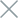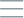• Home
• Tags
• Series
•# State-Based Search Algorithm in PHP: Explanation & Example

The State-Based Search algorithm is a crucial technique in PHP programming, employed to find solutions in problems with a state-based nature. This algorithm is often utilized in tasks such as finding paths, optimization, and resource management.

## How State-Based Search Algorithm Works

The State-Based Search algorithm focuses on identifying and simulating different states of a problem. It involves the following steps:

1. Identify Initial State: The algorithm begins with the initial state of the problem.
2. Enumerate Actions: Based on the current state, the algorithm enumerates all possible actions that can be taken from that state.
3. Apply Actions: The algorithm performs actions from the current state and transitions to new states.
4. Check Termination Condition: The algorithm checks if the termination state has been reached. If not, it returns to step 2.

• Suitable for State-Based Problems: The algorithm is suitable for problems where solutions change based on different states.
• Efficient for Small Problems: With a small number of states and actions, the algorithm can efficiently search for a solution.

• Increased Computational Cost: For large problems, the algorithm may require significant computational time to create states and determine actions.
• Problem Complexity: The algorithm may face difficulties when dealing with problems that involve a large number of states and actions.

## Example and Explanation

Consider a problem of finding the shortest path from point A to point B on a map. The Dijkstra's algorithm, a state-based search algorithm, can be employed to solve this problem efficiently.

``````\$graph = array(
'A' => array('B' => 5, 'C' => 3),
'B' => array('A' => 5, 'C' => 2, 'D' => 4),
'C' => array('A' => 3, 'B' => 2, 'D' => 7),
'D' => array('B' => 4, 'C' => 7)
);

function dijkstra(\$graph, \$start, \$end) {
// Implementation of Dijkstra's algorithm
// ...
}

\$startNode = 'A';
\$endNode = 'D';

\$shortestPath = dijkstra(\$graph, \$startNode, \$endNode);
if (empty(\$shortestPath)) {
echo "No path found from \$startNode to \$endNode.";
} else {
\$pathString = implode(' -> ', \$shortestPath);
echo "Shortest path from \$startNode to \$endNode: \$pathString.";
}
``````

In this example, the Dijkstra's algorithm utilizes a state-based search approach to find the shortest path from point A to point D on a given map. The algorithm identifies states (points) and actions (path segments) to generate the shortest path. The result is presented as a list of points along the shortest path.

While this example showcases how the state-based search algorithm can be used to solve the shortest path problem, this algorithm can also be applied to various other problems in PHP, such as game planning, task scheduling, and decision-making.